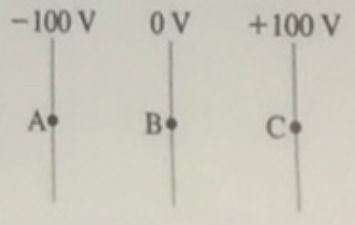# Problem: Part A. A proton is released from rest at point b, where the potential is 0 V. Afterward, the proton(A) moves toward A with a steady speed(B) moves toward A with an increasing speed(C) moves toward C with a steady speed(D) moves toward C with an increasing speed(E) remains at rest at BPart B. The potential at the metal plate A (cathode) is -100 V while that of metal plate C (anode) is +100V. The distance between the two plates is 5.0 mm. Determine the electric field at point B, where the potential is 0 V

###### FREE Expert Solution

In this problem, we are dealing with the motion of charges and the electric field.

Electric field:

83% (158 ratings)###### Problem Details

Part A. A proton is released from rest at point b, where the potential is 0 V.Afterward, the proton

(A) moves toward A with a steady speed

(B) moves toward A with an increasing speed

(C) moves toward C with a steady speed

(D) moves toward C with an increasing speed

(E) remains at rest at B

Part B. The potential at the metal plate A (cathode) is -100 V while that of metal plate C (anode) is +100V. The distance between the two plates is 5.0 mm. Determine the electric field at point B, where the potential is 0 V